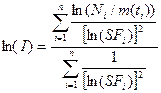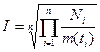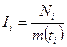In this stage, a simple evaluation is carried out using parameter values chosen a priori: before the evaluation is carried out. The procedure is very similar to the “Standard procedure” (Stage 3). The main difference is that in a special procedure there should be more than one measurement.

Step 5.1 : Identification and preparation of measurement data. It is expected that there will be more than one measurement available for a special assessment (Mi for i = 1 to n). It is therefore important that realistic uncertainties are assigned to the data (“scattering factor”, SF, Step 2.1) There may be more than one type of measurement (urine, faeces, etc), and there may be measurements of more than one radionuclide involved in the exposure. If a specific incident (and hence time of intake) was not identified, the results of workplace monitoring, such as personal or room air sampling, should be checked to give guidance on the time course of intake.

Step 5.2 : (As Step 2.3 for a single measurement.) The contributions (Pi) from all previous intakes of the radionuclide considered are calculated, taking into account all pathways of intake, and all intakes of mixtures where the radionuclide was involved. The net values (Ni = Mi – Pi) of the radionuclide are calculated by subtracting Pi from the measured values Mi.

Step 5.3 : (As Step 3.2 in the Standard Procedure, Stage 3, except for time of intake). Case or site specific parameter values should be assigned as far as they are available. Such a priori information needs to be well established and documented. Examples might include the Activity Median Aerodynamic Diameter, AMAD, (if it has been determined by appropriate air sampling, e.g., cascade impactor), specific absorption parameter values (if the inhaled material is sufficiently well characterised), or the time of intake (if potential exposure was limited, or an incident was known to occur). Otherwise the following default parameter values should be used:

• Mode of intake: Single intake
• Absorption Type and f1 value: defaults according to ICRP Publication 68, Annexe F (see Table 3.3). If the compound is unknown, then for those elements where there is a choice of absorption Types, the Type for “unspecified compounds” should be used, if available. For uranium, “unspecified compounds” are not listed, and it is proposed that Type M is assumed in the absence of specific information, as in ICRP Publication 71 (1995).

Step 5.4 : Time of intake known/unknown. If the special procedure was initiated as a result of a known incident (and hence the time of intake is known) then a simple assessment (Step 5.5) should be carried out which is consistent with the Standard evaluation (Stage 3). If the special procedure was initiated as a result of a routine measurement being inconsistent with previous assessment (Step 2.6) or a dose >1 mSv resulting from the Standard evaluation (Step 3.4) where the time of intake is probably not known, then further special procedures (Stage 5B) are needed for more detailed evaluation of the case.

Step 5.5 : (As step 3.3 in the Standard Procedure, Stage 3, but for more than one measurement). Using the assigned a priori parameter values, an estimate of intake Ii is obtained by dividing the net measured value Ni = Mi – Pi by the appropriate retention or excretion function mi(t). The best estimate of intake can be calculated according to Section 3 with the equation:where SFi is the scattering factor of the net measured value Ni . If the scattering factor is the same for all measurements, the equation results ini.e. the best estimate of the intake is the geometric mean of the intakescalculated from the single measurements. Using the same assigned a priori parameter values the committed effective dose is calculated by multiplying the “best estimate” of intake by the appropriate dose coefficient (dose per unit intake).

Step 5.6 : If the effective dose estimated in step 5.5 (taking into account all available monitoring data) is less than 1 mSv, there is no need for further investigation (Step 5.6.1). (The dose from the intake under consideration, rather than the “annual dose” as in Step 3.4, is the criterion, because intakes requiring special assessment procedures should be unusual for any individual worker.) Otherwise further special procedures (Stage 5B) are needed for more detailed evaluation of the case. Caution must be taken when the default absorption type does not lead to a conservative estimate of the dose, for example in the case of Sr.

Step 5.6.1 : The results in terms of intake and committed effective dose from Step 5.6 are recorded together with the corresponding parameter values from Step 5.3.

Prof. Dr.-Ing. Hans Richard Doerfel

IDEA System GmbH, Am Burgweg 4, D-76227 Karlsruhe, Germany.

E-Mail: info@idea-system.com